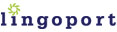# Locale-Sensitive Java Method

### `java.lang.Double`

`public static double parseDouble(String s)`

### Internationalization (I18n) Method Overview

The `parseDouble` method returns a `double` primitive value by parsing a floating point string.

### I18n Issues

The problem with calling `parseDouble` is not locale aware. For example, if a string is set to `"2,3"` where a comma is used as the decimal separator, the `parseDouble` method will not create the correct `double` primitive value. This is because it is designed to assume that a period will be used as the decimal separator.

### Suggested Replacement

To ensure that locale is applied when converting from double strings to double values, use the `DecimalFormat` class.

```double myDouble = Double.parseDouble(myDecimalString); ```

Use:

``` double myDouble = 0; //Retrieve the runtime user's locale Locale locale = getUserLocale(); //Now call the NumberFormat factory method //and pass the locale object NumberFormat f = NumberFormat.getInstance(locale); //If it is in fact an instance of DecimalFormat, //cast it as such and use it as needed if (f instanceof DecimalFormat) {     try {         myDouble = ((DecimalFormat) f).parse(myDecimalString).doubleValue();     } catch (ParseException e) {} } ```